# Fundamental Analysis of Sirius XM (part 2)

Jul. 15, 2011 8:46 AM ETSIRI
Please Note: Blog posts are not selected, edited or screened by Seeking Alpha editors.

Value, Special Situations, Long-Term Horizon, Contrarian

Contributor Since 2010

Special interest in marketplace companies, exchanges and other "croupiers".

Focus on software and travel industry.

See part 1

This article is the second part of my analysis of Sirius XM. In the first part it was conjured that cash flow is the single most important performance metric of the company. The most important aspect of the business model is the operational leverage, as fixed costs are high and variable costs are low. Any valuation approach would have to take that effect into account.

As EBITDA margin will be a lagging variable in the model as it increases incrementally. The company indicates that at maturity EBITDA margins are over 40%.

Methodology

Before I begin I would like to clarify a few aspects of the valuation. As I want to keep the model simple, with the main emphasis on sensitivity, I use a perpetual model with no forecasting period. I realize that by not using a forecasting period I am making a certain trade-off as the model will not take into account the satellite network maintenance cycle.

My aim is to make a static representation of possible owner earnings/cash flow from the current business model. It should answer the question: what kind of earnings could SIRI attain with its current platform? Consequently, this means that the model will not account for growth as the assumption is that growth will come through additional investment and how management will allocate capital in the future is not relevant to current valuation.  A scenario analysis with three scenarios will be presented: at maturity (best case), current status and a shock scenario (worst case).

The key drivers of Sirius XM value are:

-         Subscriber growth.

-         Average Revenue Per User (ARPU)

-         Variable costs

-         Required return

Table 1: General Assumptions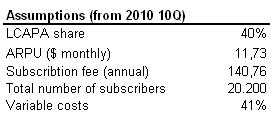Estimating the state of maturity

In its presentations, the company indicates that “at maturity” EBITDA margins will come in at over 40%. We know that this scalable effect is caused by operational leverage as fixed costs are high, but we do not know how this state of maturity looks like.

The companies income statement was reformulated to come up with an estimate on the break-up between fixed and variable costs. According to this exercise, variable costs are around 41-49% of revenue and fixed cost are about \$900 million per year (see figure 1 and 2). The adjusted EBITDA for 2010 according to the reformulated income statement was 26.2%

Figure 1: Reformulated balance sheet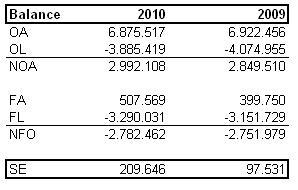Figure 2: Reformulated income statement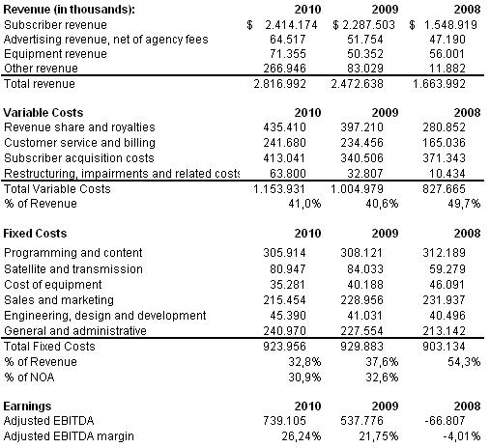Knowing subscriber levels in 2010 were 20.2 million, the corresponding adjusted EBITDA margins and estimating the fixed and variable costs inputs, we can make an assumption on what the state of maturity looks like in terms of subscribers and ARPU (see figure 3).

Figure 3: an estimate of the state of maturity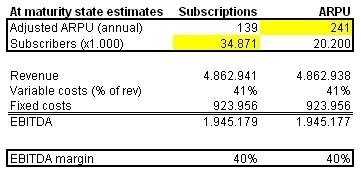Required return

The required return investment (equity) was 25%. I use the senior notes due in 2018, issued in October 2010 as a proxy to determine long term debt financing cost, at 7,625%. After adjusting the balance sheet I determine that Net Financial Obligations are 93% of total financing (see figure 4). Therefore, required return of Net Operating Assets is 8.87%, which corresponds to an EV/EBITDA multiple of 11.28.

Figure 4: Required Return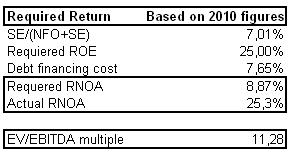Assigned probabilities

This is a complete guesswork. I assume that shocks are unlikely with a probability of 1 out of 10. The current state represents 50% of the valuation and the maturity state 40%.

Scenario analysis

According to this valuation, common shares of Sirius XM are fairly valued at \$1.54. In the current state perpetuity the value was \$0.85 per share, -\$0.45 in the shock scenario and \$2.92 in the state of maturity scenario.

Figure 5: Scenario Analysis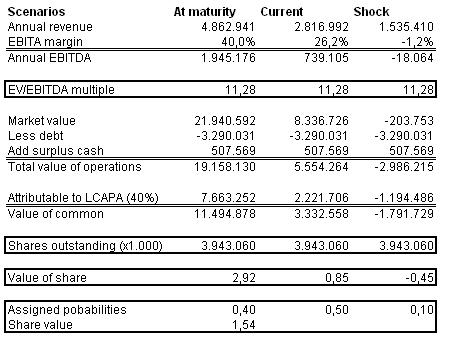Sensitivity of key value drivers

To examine how the value drivers affect the valuation, a sensitivity analysis was performed on all for drivers (see figure 7). Subscriber levels are a very sensitive driver of value as an increase of 5 million (~25%) in the subscriber base, cause an increase in per share value of 82% in the model. ARPU is also very sensitive as a 17% increase will cause a 59% increase in per share value in the model. By lowering variable costs by 2.5 percentage points (to 38.5%), value of shares will increase by 14% in the model. The EV/EBITDA seems to be a fairly sensitive driver, as a lowered required return on net operating assets of 7.53% would increase the valuation by 26%.

Figure 6: Sensitivity Analysis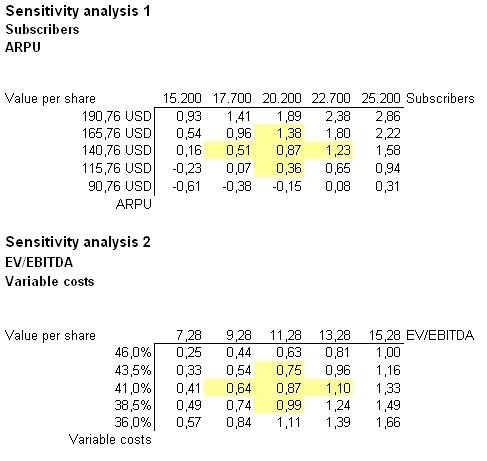In part three I try a different valuation approach by a applying a Monte Carlo simulation to probable earning outcomes of Sirius XM’s current business model.

Continue to part 3

Disclosure: I have no positions in any stocks mentioned, and no plans to initiate any positions within the next 72 hours.